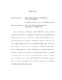## Exponentiation of Motivic Zeta Functions2017
##### Authors
Huang, Jonathan Andrew
We provide a formula for the generating series of the Weil zeta function $Z(X,t)$ of symmetric powers $\Sym^n X$ of varieties $X$ over finite fields. This realizes the zeta function $Z(X,t)$ as an exponentiable measure whose associated Kapranov motivic zeta function takes values in $W(R)$ the big Witt ring of $R=W(\Z)$. We apply our formula to compute $Z(\Sym^n X,t)$ in a number of explicit cases. Any motivic zeta function $\zeta_\mu$ of a measure $\mu$ factoring through the Grothendieck ring of Chow motives is itself exponentiable; in fact, this applies to $\zeta_\mu$ as a motivic measure itself. We prove a condition for which any motivic measure taking values in a $\lambda$-ring has an associated motivic zeta function $Z = \zeta_\mu$ that is itself an exponentiable measure $\mu_Z = Z$, and this process is shown to iterate indefinitely. This involves a study of the case of $\lambda$-ring-valued motivic measures. Finally, we provide an understanding of MacDonald's formula in this context.